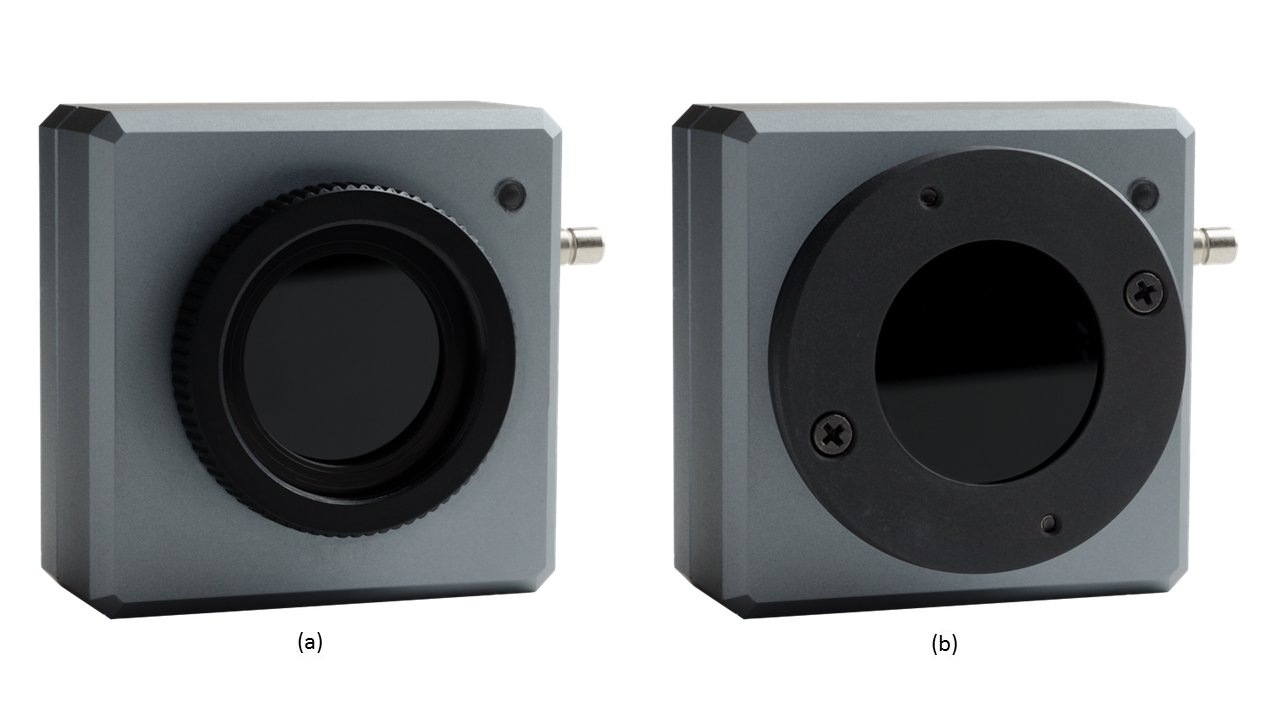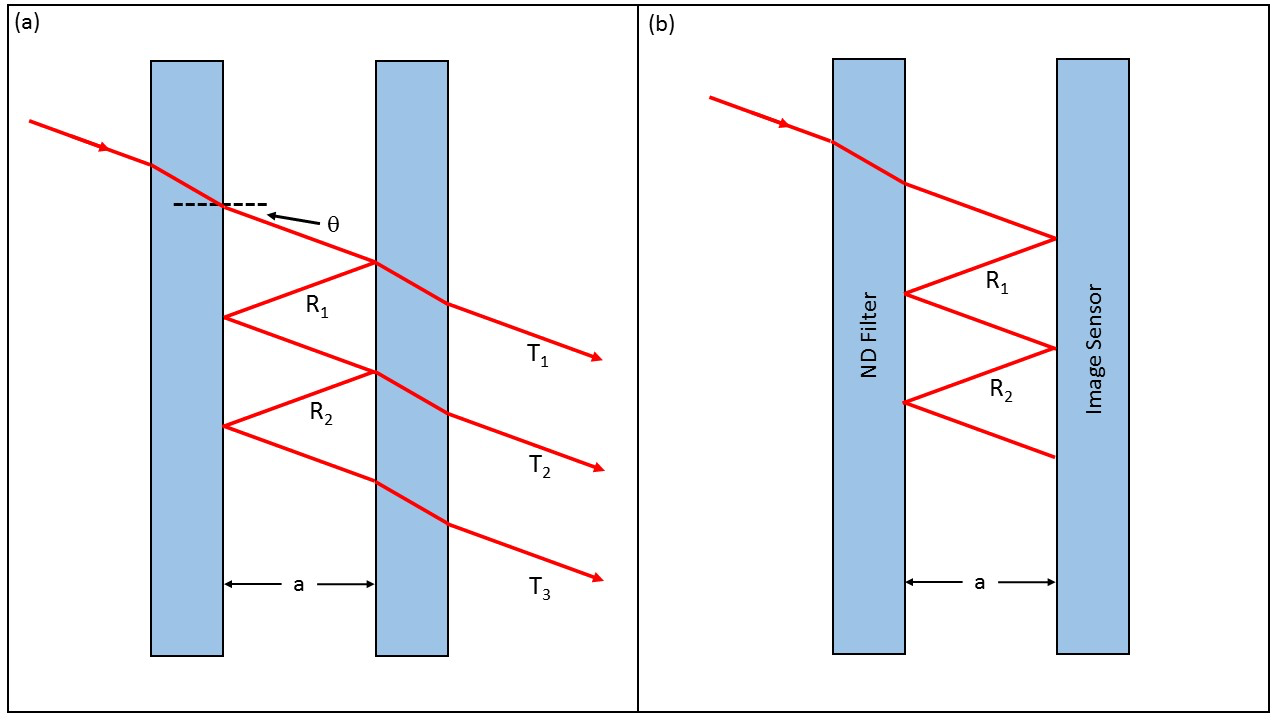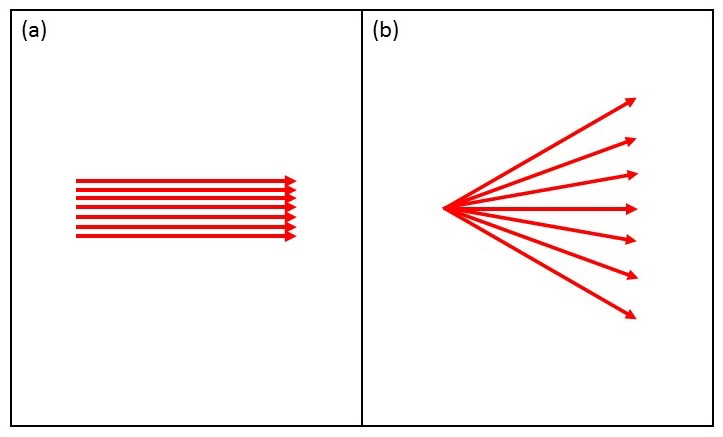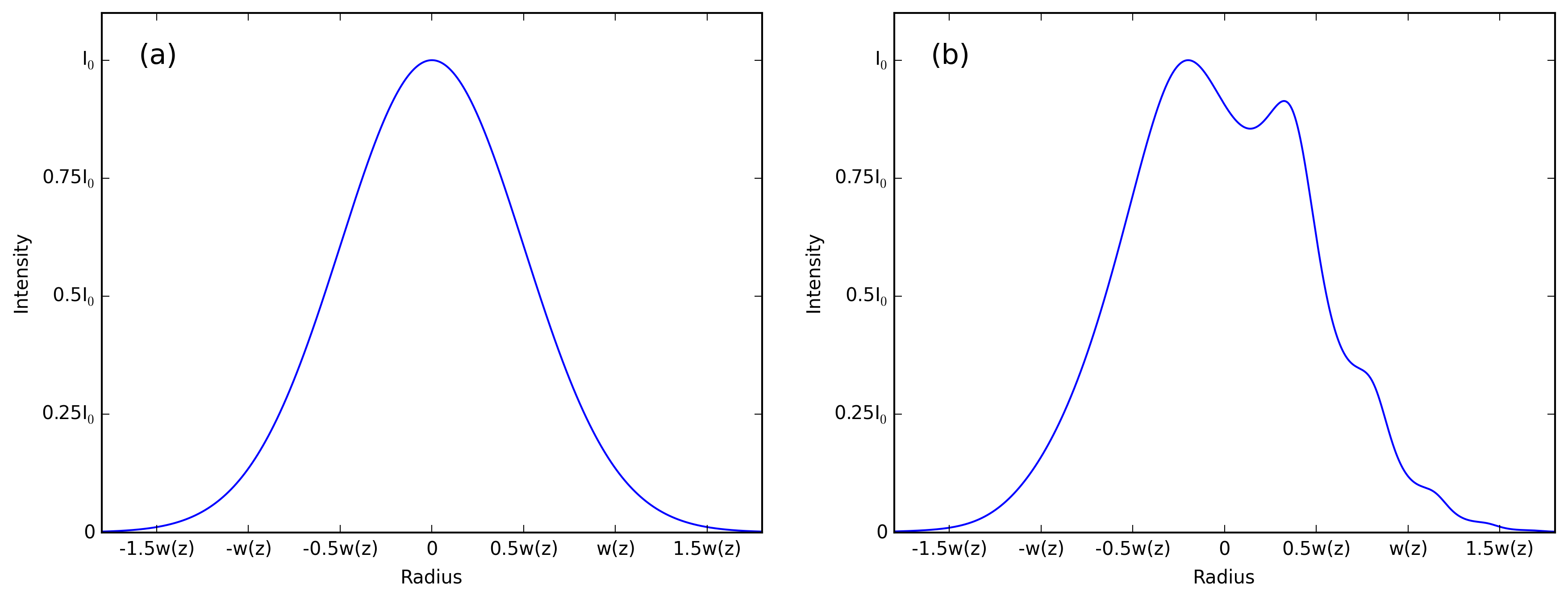July 21, 2016

# Why We Tilt Our Filters

#### Introduction

DataRay’s beam profiling cameras are made to be used with Neutral Density (ND) filters which provide attenuation in an effort to avoid over saturation of the image sensor’s pixels. Each of DataRay’s cameras are shipped with a set of three ND filters&dash;either MagND or C-mount. DataRay’s MagND filters are attached to the WinCamD-LCM4 (see Fig. 1b) with magnets, and the filters magnetically stack so that higher attenuation can be achieved through use of multiple ND filters. DataRay’s WinCamD series cameras are compatible with the C-mount ND filters (see Fig. 1a). The C-mount is a standardized mount, allowing the ND filters to be used with other accessories. Like the MagND filters, the C-mount filters are stackable (providing higher attenuation) with both male and female threads on each filter. An important feature of both the MagND and C-mount filters is their orientation relative to the surface of the imaging sensor. In this blog post, we explain why tilting our filters is necessary for an accurate beam measurement.Figure 1: (a) C-Mount ND filter attached to a WinCamD-LCM4 The ND C-Mount filters are set at a 3$$^\circ$$ in their case to prevent reflection and interference effects. (b) MagND filter attached to a WinCamD-LCM4. The MagND filters are set at a 2$$^\circ$$ in their respective cases to prevent reflection and interference effects.

#### Optical Feedback

Optical feedback occurs when light is reflected back into the laser cavity and has been shown to cause changes in the gain, and output spectrum. If the ND filter attached to the camera is not tilted, then the laser beam can be reflected back to the source (see Fig. 2). Therefore, to avoid optical feedback and its deleterious effects, DataRay's filters are set at an angle in their housings.Figure 2: (a) Shows a beam (red arrows) reflected from the ND filter back to the source. (b) A beam (red arrows) reflected from the ND filter and the image sensor; however, the ND filter is tilted so that the reflected beam does not strike the source.

#### Internal Reflection and InterferenceFigure 3: (a) An Fabry-Perot interferometer comprised of two mirrors (blue rectangles) with a ray of light (red line/arrows) undergoing multiple reflections. The transmitted rays are summed together in a geometric series which yields Eq. 1. (b) Ray of light reflected between the ND filter and image sensor can be roughly modeled by the Fabry-Perot interferometer in (a).

As the beam strikes the sensor surface, a majority of the light will be absorbed by the silicon detector, but a small percentage will be reflected back toward the ND filter. A percentage of the light will pass through the filter and be transmitted, but, again, a portion will be reflected back to the sensor. This process will continue ad infintum so that the intensity distribution returned by the sensor is in actuality a summation of the initial beam and the internal reflections. The percentage of light reflected each time the light strikes a surface is dictated by the Fresnel equations which relate the percentage of reflected light to the material's index of refraction and the beam's incident angle.Figure 4: (a) A collimated beam striking the surface of the Fabry-Perot interferometer would produce a uniformly modulated beam (see Fig. 5a) (b) In reality, beams are slightly divergent which results in an interference pattern (see Fig. 5b).Figure 5: (a) Gaussian beam’s time-averaged intensity distribution as a function of radius. (b) Gaussian beam’s time-averaged intensity distribution as a function of radius, but displaying the interference pattern that occurs for a Fabry-Perot interferometer. This type of pattern can occur if the ND filters are parallel with the image sensor.

Making the assumption that the ND filter and the sensor chip have the same index of refraction allows us to use the Fabry-Perot interferometer as an example of internal reflections and the interference (see Fig. 3). Light enters the interferometer and is reflected between two mirrors with the same index of refraction (see Fig.3a). The beams exiting the interferometer constructively or destructively interfere so that the summation of the individual transmissions is given by a known geometric series. The overall transmission function $$T_e$$ is

\begin{equation} T_e=\frac{T^2}{1+R^2-2R\cos\delta} \label{intensity} \end{equation}

where $$R$$ is the reflectance given by the Fresnel equations and $$T=1-R$$. The path length difference $$\delta$$ is given by

\begin{equation} \delta=2ka\cos\theta \end{equation}

where $$a$$ is the distance separating the two mirrors, $$k$$ is the wave number, and $$\theta$$ is the angle of the light as it exits the first mirror (see Fig. 3a). Equation 1 gives the change in intensity for light passing through the interferometer at angle $$\theta$$ (see Fig. 4a). However, a real laser beam is slightly divergent so that all the rays enter the interferometer with a different angle (see Fig. 4b). In this case, the rays will have separately modulated intensities and an interference pattern will be seen in the beam (see Fig. 5b). Therefore, the ND filter is set at an angle to the sensor, noticeably reducing the interference pattern and increasing the accuracy of measurements. Wedge filters are used to a similar effect in some optical systems.

#### Application Note

An application note covering this topic is available here.

#### Conclusion

DataRay's MagND and C-mount ND filters are slightly tilted to avoid optical feedback and interference. If you have any questions about beam attenuation or laser beam profiling, please contact us. We have years of experience with laser profiling and would love to find a solution for your system. Visit our website to see the various beam attenuation options offered by DataRay, as well as our full line of beam profiling cameras.

Posted by: Lucas Hofer